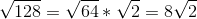## Example Questions

### Example Question #1011 : Sat Mathematics

Simplify: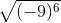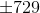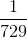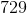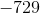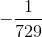Explanation:

To solve this problem, consider PEMDAS first. Do the math inside the parentheses before performing the square root.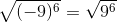Then we just find perfect squares inside the radical.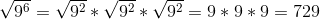Square roots will always generate real values.

### Example Question #62 : Basic Squaring / Square Roots

Simplify: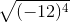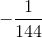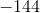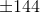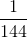Explanation:

To solve this problem, consider PEMDAS first. Do the math inside the parentheses before performing the square root.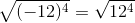Then we just find perfect squares inside the radical.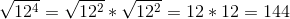Square roots will always generate real values.

### Example Question #61 : Basic Squaring / Square Roots

Simplify: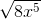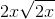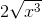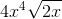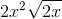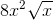Explanation:

To simplify radicals, we need to find a perfect square to factor out. In this case, its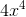.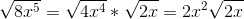### Example Question #66 : Basic Squaring / Square Roots

Simplify: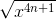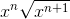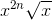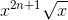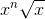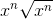Explanation:

We need to apply the power rule of multiplying exponents and break it down.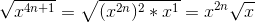### Example Question #67 : Basic Squaring / Square Roots

Simplify: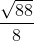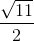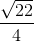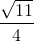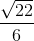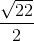Explanation:

To simplify radicals, we need to find a perfect square to factor out. In this case, its.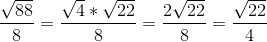### Example Question #31 : How To Simplify Square Roots

Simplify: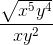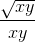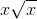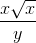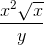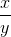Explanation:

To simplify radicals, we need to find a perfect square to factor out. In this case, its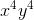.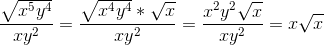### Example Question #131 : Arithmetic

Simplify: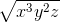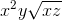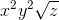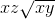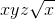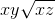Explanation:

To simplify radicals, we need to find a perfect square to factor out. In this case, its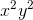.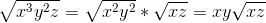### Example Question #64 : Basic Squaring / Square Roots

Simplify: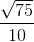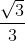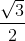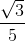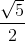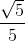Explanation:

To simplify a square root, we need to find perfect squares. In this case, it is.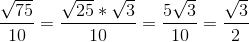Remember you can reduce the fraction even further.

### Example Question #71 : Basic Squaring / Square Roots

Simplify: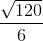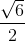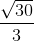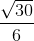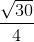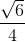Explanation:

To simplify a square root, we need to find perfect squares. In this case, it is.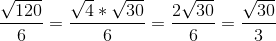Remember you can reduce the fraction even further.

### Example Question #72 : Basic Squaring / Square Roots

Simplify: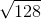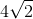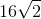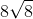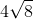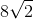To simplify radicals, we need to factor out perfect squares. In this case, it's.